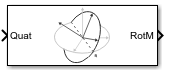# Coordinate Transformation Conversion

Convert to a specified coordinate transformation representation

• Library:
• Robotics System Toolbox / Utilities

ROS Toolbox / Utilities

UAV Toolbox / Utilities

•## Description

The Coordinate Transformation Conversion block converts a coordinate transformation from the input representation to a specified output representation. The input and output representations use the following forms:

• Axis-Angle (`AxAng`) – ```[x y z theta]```

• Euler Angles (`Eul`) – `[z y x]`, `[z y z]`, or `[x y z]`

• Homogeneous Transformation (`TForm`) – 4-by-4 matrix

• Quaternion (`Quat`) – `[w x y z]`

• Rotation Matrix (`RotM`) – 3-by-3 matrix

• Translation Vector (`TrVec`) – ```[x y z]```

All vectors must be column vectors.

To accommodate representations that only contain position or orientation information (`TrVec` or `Eul`, for example), you can specify two inputs or outputs to handle all transformation information. When you select the Homogeneous Transformation as an input or output, an optional ```Show TrVec input/output port``` parameter can be selected on the block mask to toggle the multiple ports.

## Ports

### Input

expand all

Input transformation, specified as a coordinate transformation. The following representations are supported:

• Axis-Angle (`AxAng`) – ```[x y z theta]```

• Euler Angles (`Eul`) – ```[z y x]```, `[z y z]`, or ```[x y z]```

• Homogeneous Transformation (`TForm`) – 4-by-4 matrix

• Quaternion (`Quat`) – ```[w x y z]```

• Rotation Matrix (`RotM`) – 3-by-3 matrix

• Translation Vector (`TrVec`) – `[x y z]`

All vectors must be column vectors.

To accommodate representations that only contain position or orientation information (`TrVec` or `Eul`, for example), you can specify two inputs or outputs to handle all transformation information. When you select the Homogeneous Transformation as an input or output, an optional `Show TrVec input/output port` parameter can be selected on the block mask to toggle the multiple ports.

Translation vector, specified as a 3-element column vector, `[x y z]`, which corresponds to a translation in the x, y, and z axes respectively. This port can be used to input or output the translation information separately from the rotation vector.

#### Dependencies

You must select Homogeneous Transformation (`TForm`) for the opposite transformation port to get the option to show the additional `TrVec` port. Enable the port by clicking ```Show TrVec input/output port```.

### Output Arguments

expand all

Output transformation, returned as a coordinate transformation with the specified representation. The following representations are supported:

• Axis-Angle (`AxAng`) – ```[x y z theta]```

• Euler Angles (`Eul`) – ```[z y x]```, `[z y z]`, or ```[x y z]```

• Homogeneous Transformation (`TForm`) – 4-by-4 matrix

• Quaternion (`Quat`) – ```[w x y z]```

• Rotation Matrix (`RotM`) – 3-by-3 matrix

• Translation Vector (`TrVec`) – `[x y z]`

To accommodate representations that only contain position or orientation information (`TrVec` or `Eul`, for example), you can specify two inputs or outputs to handle all transformation information. When you select the Homogeneous Transformation as an input or output, an optional `Show TrVec input/output port` parameter can be selected on the block mask to toggle the multiple ports.

Translation vector, returned as a three-element column vector, `[x y z]`, which corresponds to a translation in the x, y, and z axes respectively. This port can be used to input or output the translation information separately from the rotation vector.

#### Dependencies

You must select Homogeneous Transformation (`TForm`) for the opposite transformation port to get the option to show the additional `TrVec` port. Enable the port by clicking ```Show TrVec input/output port```.

## Parameters

expand all

Select the representation for both the input and output port for the block. If you are using a transformation with only orientation information, you can also select the `Show TrVec input/output port` when converting to or from a homogeneous transformation.

Order of the Euler angle axis rotations, specified as `ZYX`, `ZYZ`, or `XYZ`. The order of the angles in the input or output port `Eul` must match this rotation sequence. The default order `ZYX` specifies an orientation by:

• Rotating about the initial z-axis

• Rotating about the intermediate y-axis

• Rotating about the second intermediate x-axis

#### Dependencies

You must select `Euler Angles` for the `Representation` input or output parameter. The axis rotation sequence only applies to Euler angle rotations.

Toggle the `TrVec` input or output port when you want to specify or receive a separate translation vector for position information along with an orientation representation.

#### Dependencies

You must select Homogeneous Transformation (`TForm`) for the opposite transformation port to get the option to show the additional `TrVec` port.

• `Interpreted execution` — Simulate model using the MATLAB® interpreter. This option shortens startup time but has a slower simulation speed than ```Code generation```. In this mode, you can debug the source code of the block.

• `Code generation` — Simulate model using generated C code. The first time you run a simulation, Simulink® generates C code for the block. The C code is reused for subsequent simulations, as long as the model does not change. This option requires additional startup time, but the speed of the subsequent simulations is comparable to ```Interpreted execution```.

Tunable: No

## Version History

Introduced in R2017b